# In-Place Algorithm

In-place has more than one definitions. One strict definition is.

An in-place algorithm is an algorithm that does not need an extra space and produces an output in the same memory that contains the data by transforming the input ‘in-place’. However, a small constant extra space used for variables is allowed.

In-place means that the algorithm does not use extra space for manipulating the input but may require a small though nonconstant extra space for its operation. Usually, this space is O(log n), though sometimes anything in o(n) (Smaller than linear) is allowed [Source : Wikipedia]

A Not In-Place Implementation of reversing an array

## C++

 `// An Not in-place C++ program to reverse an array ` `#include ` `using` `namespace` `std; ` `  `  `/* Function to reverse arr[] from start to end*/` `void` `revereseArray(``int` `arr[], ``int` `n) ` `{ ` `   ``// Create a copy array and store reversed ` `   ``// elements ` `   ``int` `rev[n]; ` `   ``for` `(``int` `i=0; i

## Java

 `// An Not in-place Java program ` `// to reverse an array ` `import` `java.util.*; ` ` `  `class` `GFG ` `{ ` `    ``/* Function to reverse arr[] ` `       ``from start to end*/` `    ``public` `static` `void` `revereseArray(``int` `[]arr,  ` `                                     ``int` `n) ` `    ``{ ` `        ``// Create a copy array ` `        ``// and store reversed ` `        ``// elements ` `        ``int` `[]rev = ``new` `int``[n]; ` `        ``for` `(``int` `i = ``0``; i < n; i++) ` `            ``rev[n - i - ``1``] = arr[i]; ` `         `  `        ``// Now copy reversed  ` `        ``// elements back to arr[] ` `        ``for` `(``int` `i = ``0``; i < n; i++) ` `            ``arr[i] = rev[i]; ` `    ``}  ` `     `  `    ``/* Utility function to ` `       ``print an array */` `    ``public` `static` `void` `printArray(``int` `[]arr,  ` `                                  ``int` `size) ` `    ``{ ` `    ``for` `(``int` `i = ``0``; i < size; i++) ` `        ``System.out.print(arr[i] + ``" "``); ` `    ``System.out.println(``""``); ` `    ``}  ` `     `  `    ``// Driver code ` `    ``public` `static` `void` `main(String[] args)  ` `    ``{ ` `        ``int` `arr[] = {``1``, ``2``, ``3``, ``4``, ``5``, ``6``};  ` `        ``int` `n = arr.length; ` `        ``printArray(arr, n);  ` `        ``revereseArray(arr, n);  ` `        ``System.out.println(``"Reversed array is"``); ` `        ``printArray(arr, n);  ` `    ``} ` `} ` ` `  `// This code is contributed ` `// by Harshit Saini `

## Python3

 `# An Not in-place Python program  ` `# to reverse an array ` ` `  `''' Function to reverse arr[] ` `    ``from start to end '''` `def` `revereseArray(arr, n): ` `     `  `    ``# Create a copy array  ` `    ``# and store reversed ` `    ``# elements ` `    ``rev ``=` `n ``*` `[``0``] ` `    ``for` `i ``in` `range``(``0``, n): ` `        ``rev[n ``-` `i ``-` `1``] ``=` `arr[i] ` `             `  `    ``# Now copy reversed ` `    ``# elements back to arr[] ` `    ``for` `i ``in` `range``(``0``, n): ` `        ``arr[i] ``=` `rev[i] ` `         `  `# Driver code ` `if` `__name__ ``=``=` `"__main__"``: ` `    ``arr ``=` `[``1``, ``2``, ``3``, ``4``, ``5``, ``6``] ` `    ``n ``=` `len``(arr) ` `    ``print``(``*``arr)  ` `    ``revereseArray(arr, n);  ` `    ``print``(``"Reversed array is"``) ` `    ``print``(``*``arr)  ` `     `  `# This code is contributed ` `# by Harshit Saini `

## C#

 `// An Not in-place C# program ` `// to reverse an array ` `using` `System; ` ` `  `class` `GFG ` `{ ` `    ``/* Function to reverse arr[] ` `    ``from start to end*/` `    ``public` `static` `void` `revereseArray(``int``[] arr,  ` `                                    ``int` `n) ` `    ``{ ` `        ``// Create a copy array ` `        ``// and store reversed ` `        ``// elements ` `        ``int``[] rev = ``new` `int``[n]; ` `        ``for` `(``int` `i = 0; i < n; i++) ` `            ``rev[n - i - 1] = arr[i]; ` `         `  `        ``// Now copy reversed  ` `        ``// elements back to arr[] ` `        ``for` `(``int` `i = 0; i < n; i++) ` `            ``arr[i] = rev[i]; ` `    ``}  ` `     `  `    ``/* Utility function to ` `    ``print an array */` `    ``public` `static` `void` `printArray(``int``[] arr,  ` `                                ``int` `size) ` `    ``{ ` `    ``for` `(``int` `i = 0; i < size; i++) ` `        ``Console.Write(arr[i] + ``" "``); ` `    ``Console.Write(``"\n"``); ` `    ``}  ` `     `  `    ``// Driver code ` `    ``public` `static` `void` `Main()  ` `    ``{ ` `        ``int``[] arr = {1, 2, 3, 4, 5, 6};  ` `        ``int` `n = arr.Length; ` `        ``printArray(arr, n);  ` `        ``revereseArray(arr, n);  ` `        ``Console.WriteLine(``"Reversed array is"``); ` `        ``printArray(arr, n);  ` `    ``} ` `} ` ` `  `// This code is contributed by Ita_c. `

Output:

```1 2 3 4 5 6
Reversed array is
6 5 4 3 2 1
```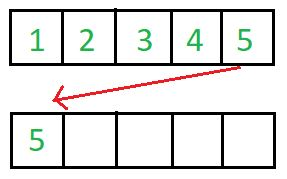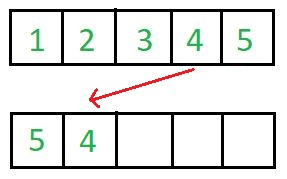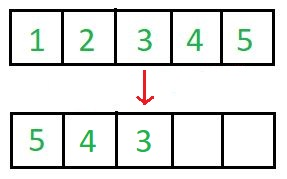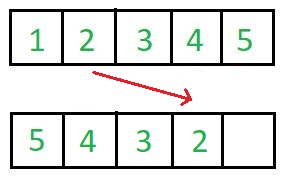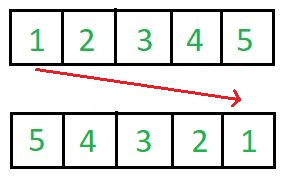This needs O(n) extra space and is an example of not-in-place algorithm.

An In-Place Implementation of Reversing an array.

## C++

 `// An in-place C++ program to reverse an array ` `#include ` `using` `namespace` `std; ` `  `  `/* Function to reverse arr[] from start to end*/` `void` `revereseArray(``int` `arr[], ``int` `n) ` `{ ` `   ``for` `(``int` `i=0; i

## Java

 `// An in-place Java program ` `// to reverse an array ` `import` `java.util.*; ` ` `  `class` `GFG ` `{ ` `    ``public` `static` `int` `__(``int` `x, ``int` `y) {``return` `x;} ` `     `  `    ``/* Function to reverse arr[]  ` `       ``from start to end*/` `    ``public` `static` `void` `revereseArray(``int` `[]arr,  ` `                                     ``int` `n) ` `    ``{ ` `        ``for` `(``int` `i = ``0``; i < n / ``2``; i++) ` `            ``arr[i] = __(arr[n - i - ``1``],  ` `                        ``arr[n - i - ``1``] = arr[i]); ` `    ``}  ` `     `  `    ``/* Utility function to  ` `       ``print an array */` `    ``public` `static` `void` `printArray(``int` `[]arr,  ` `                                  ``int` `size) ` `    ``{ ` `        ``for` `(``int` `i = ``0``; i < size; i++) ` `            ``System.out.print(Integer.toString(arr[i]) + ``" "``);  ` `        ``System.out.println(``""``); ` `    ``}  ` `     `  `    ``// Driver code ` `    ``public` `static` `void` `main(String[] args)  ` `    ``{ ` `        ``int` `[]arr = ``new` `int``[]{``1``, ``2``, ``3``, ``4``, ``5``, ``6``};  ` `        ``int` `n = arr.length; ` `        ``printArray(arr, n);  ` `        ``revereseArray(arr, n);  ` `        ``System.out.println(``"Reversed array is"``); ` `        ``printArray(arr, n);  ` `    ``} ` `} ` ` `  `// This code is contributed  ` `// by Harshit Saini `

## Python3

 `# An in-place Python program ` `# to reverse an array ` ` `  `''' Function to reverse arr[] ` `    ``from start to end'''` `def` `revereseArray(arr, n): ` `     `  `    ``for` `i ``in` `range``(``0``, ``int``(n ``/` `2``)): ` `        ``arr[i], arr[n ``-` `i ``-` `1``] ``=` `arr[n ``-` `i ``-` `1``], arr[i] ` ` `  ` `  `# Driver code ` `if` `__name__ ``=``=` `"__main__"``: ` `     `  `    ``arr ``=` `[``1``, ``2``, ``3``, ``4``, ``5``, ``6``] ` `    ``n ``=` `len``(arr) ` `    ``print``(``*``arr) ` `    ``revereseArray(arr, n)  ` `    ``print``(``"Reversed array is"``) ` `    ``print``(``*``arr) ` `     `  `# This code is contributed  ` `# by Harshit Saini `

## C#

 `// An in-place C# program ` `// to reverse an array ` `using` `System;  ` `     `  `class` `GFG ` `{ ` `    ``public` `static` `int` `__(``int` `x, ``int` `y) {``return` `x;} ` `     `  `    ``/* Function to reverse arr[]  ` `    ``from start to end*/` `    ``public` `static` `void` `revereseArray(``int` `[]arr,  ` `                                    ``int` `n) ` `    ``{ ` `        ``for` `(``int` `i = 0; i < n / 2; i++) ` `            ``arr[i] = __(arr[n - i - 1],  ` `                        ``arr[n - i - 1] = arr[i]); ` `    ``}  ` `     `  `    ``/* Utility function to  ` `    ``print an array */` `    ``public` `static` `void` `printArray(``int` `[]arr,  ` `                                ``int` `size) ` `    ``{ ` `        ``for` `(``int` `i = 0; i < size; i++) ` `            ``Console.Write(arr[i] + ``" "``);  ` `        ``Console.WriteLine(``""``); ` `    ``}  ` `     `  `    ``// Driver code ` `    ``public` `static` `void` `Main(String[] args)  ` `    ``{ ` `        ``int` `[]arr = ``new` `int``[]{1, 2, 3, 4, 5, 6};  ` `        ``int` `n = arr.Length; ` `        ``printArray(arr, n);  ` `        ``revereseArray(arr, n);  ` `        ``Console.WriteLine(``"Reversed array is"``); ` `        ``printArray(arr, n);  ` `    ``} ` `} ` ` `  `/* This code is contributed by PrinciRaj1992 */`

Output:

```1 2 3 4 5 6
Reversed array is
6 5 4 3 2 1
```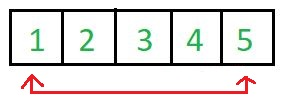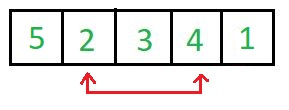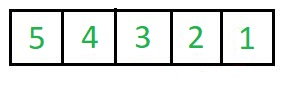This needs O(1) extra space for exchanging elements and is an example of in-place algorithm.

Which Sorting Algorithms are In-Place and which are not?
In Place : Bubble sort, Selection Sort, Insertion Sort, Heapsort.

Not In-Place : Merge Sort. Note that merge sort requires O(n) extra space.

What about QuickSort? Why is it called In-Place?
QuickSort uses extra space for recursive function calls. It is called in-place according to broad definition as extra space required is not used to manipulate input, but only for recursive calls.

Attention reader! Don’t stop learning now. Get hold of all the important DSA concepts with the DSA Self Paced Course at a student-friendly price and become industry ready.

My Personal Notes arrow_drop_upCheck out this Author's contributed articles.

If you like GeeksforGeeks and would like to contribute, you can also write an article using contribute.geeksforgeeks.org or mail your article to contribute@geeksforgeeks.org. See your article appearing on the GeeksforGeeks main page and help other Geeks.

Please Improve this article if you find anything incorrect by clicking on the "Improve Article" button below.